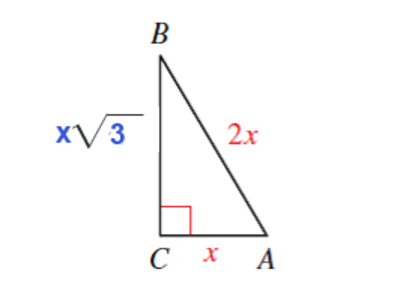## Trigonometry 7th Edition

Required trigonometric functions are- $\sin A = \frac{\sqrt 3}{2}$ $\\csc A = \frac{2}{\sqrt 3}$ $\\cos A = \frac{1}{2}$ $\\sec A = 2$ $\\tan A = \sqrt 3$ $\\\cot A = \frac{1}{\sqrt 3}$
Steps to Answer- From the given diagram of triangle ABC, we will use given information and Pythagoras Theorem to solve for 'c'- We know that - $c^{2} =a^{2} + b^{2}$ ( Pythagoras Theorem) Therefore - $a^{2} =c^{2} - b^{2}$ $a^{2} = (2x)^{2} - x^{2}$ $a^{2} =4x^{2} - x^{2}$ $a^{2} = 3 x^{2}$ therefore $a = x \sqrt 3$ Now we can write the required six T-functions of A using $a= x \sqrt 3$ ,$b = x$ and c = $2x$ $\sin A = \frac{a}{c} = \frac{x\sqrt 3}{2x} = \frac{\sqrt 3}{2}$ $\\csc A = \frac{c}{a} =\frac{2x}{x\sqrt 3} = \frac{2}{\sqrt 3}$ $\\cos A = \frac{b}{c} =\frac{x}{2x} = \frac{1}{2}$ $\\sec A = \frac{c}{b} = \frac{2x}{x} = \frac{2}{1} = 2$ $\\tan A = \frac{a}{b} = \frac{x\sqrt 3}{x} = \frac{\sqrt 3}{1} = \sqrt 3$ $\\\cot A = \frac{b}{a} = \frac{x}{x\sqrt 3} = \frac{1}{\sqrt 3}$